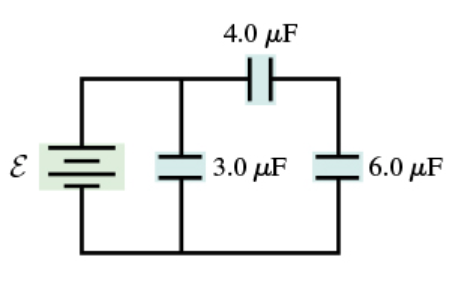# Problem: Consider the circuit shown in (Figure 1). Assume E = 16 V. What is the charge on 4.0 μF capacitor? What is the charge on 6.0 μF capacitor?

###### FREE Expert Solution

Equivalent capacitance for capacitors in series:

$\overline{)\frac{\mathbf{1}}{{\mathbf{C}}_{\mathbf{e}\mathbf{q}}}{\mathbf{=}}\frac{\mathbf{1}}{{\mathbf{C}}_{\mathbf{1}}}{\mathbf{+}}\frac{\mathbf{1}}{{\mathbf{C}}_{\mathbf{2}}}}$

The charge stored on a capacitor:

$\overline{){\mathbf{Q}}{\mathbf{=}}{\mathbf{C}}{\mathbf{V}}}$

The 4.0 μF and the 6.0 μF capacitors are in series:

C4,6 = (1/C1 + 1/C2)-1 = (1/4 + 1/6)-1 = 2.4 μF(10-6F/1μF) = 2.4 × 10-6 F

The voltage across the two capacitors is 16 V.###### Problem Details

Consider the circuit shown in (Figure 1). Assume E = 16 V. What is the charge on 4.0 μF capacitor? What is the charge on 6.0 μF capacitor?